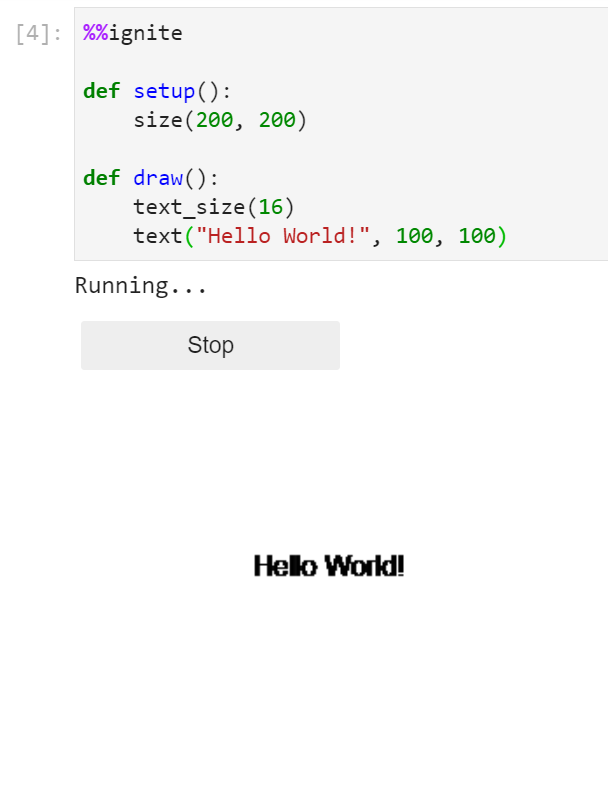# Shapes & Text

Below is a list of all of the shapes, and text available for drawing within spark.

All of the examples below assume you have the boilerplate from the notebook setup in your code

### Rectangles

To create a rectangle there are 3 options:

Function Description
rect(x, y, w, l) Draw a rectangle filled with color, and with a stroke
fill_rect(x, y, w, l) Draw an rectangle filled with a color
stroke_rect(x, y, w, l) Draw the rectangle of an ellipse (it's stroke)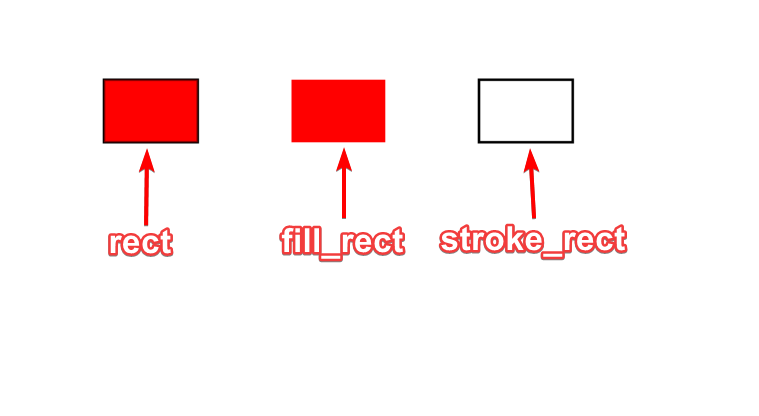Comparison of 3 functions, note the red color was added for visibility, by default fill is black

#### rect()

 `1` ``````rect(x, y, w, l) ``````

Parameters

• x: (int or float) The value of the x position of the rectangle
• y: (int or float) The value of the y position of the rectangle
• w: (int or float) The width of the rectange
• l: (int or float) The length of the rectange

Example(s):

Creating a filled and stroked rectangle at (100, 100) with a width of 75, and length of 50

 ```1 2 3 4 5 6 7``` ``````%%ignite def setup(): size(200, 200) def draw(): rect(100, 100, 75, 50) ``````

Results in: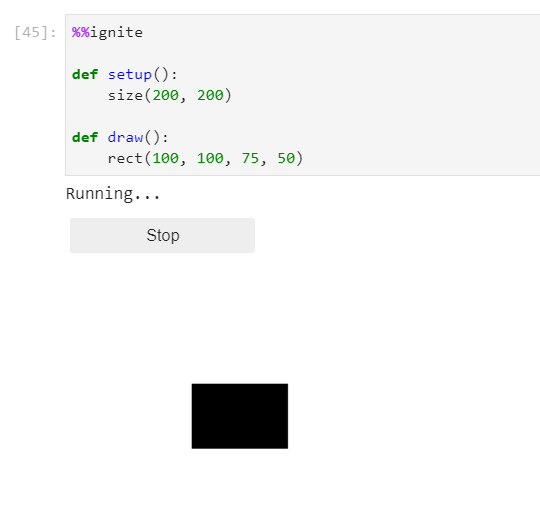#### fill_rect()

 `1` ``````fill_rect(x, y, w, l) ``````

Parameters

• x: (int or float) The value of the x position of the rectangle
• y: (int or float) The value of the y position of the rectangle
• w: (int or float) The width of the rectange
• l: (int or float) The length of the rectange

Example(s):

Creating a filled rectangle at (100, 100) with a width of 75, and length of 50

 ```1 2 3 4 5 6 7``` ``````%%ignite def setup(): size(200, 200) def draw(): fill_rect(100, 100, 75, 50) ``````

Results in:#### stroke_rect()

 `1` ``````stroke_rect(x, y, w, l) ``````

Parameters

• x: (int or float) The value of the x position of the rectangle
• y: (int or float) The value of the y position of the rectangle
• w: (int or float) The width of the rectange
• l: (int or float) The length of the rectange

Example(s):

Creating a filled rectangle at (100, 100) with a width of 75, and length of 50

 ```1 2 3 4 5 6 7``` ``````%%ignite def setup(): size(200, 200) def draw(): stroke_rect(100, 100, 75, 50) ``````

Results in:### Squares

To create a square there are 3 options:

Function Description
square(x, y, s) Draw a square filled with color, and with a stroke
fill_square(x, y, s) Draw an square filled with a color
stroke_square(x, y, s) Draw the outline of a square (its stroke)Comparison of 3 functions, note the red color was added for visibility, by default fill is black

#### square()

 `1` ``````square(x, y, s) ``````

Parameters

• x: (int or float) The value of the x position of the square
• y: (int or float) The value of the y position of the square
• s: (int or float) The width and height of the square

Example(s):

Creating a filled and stroked square at (100, 100) with a width and height of 75

 ```1 2 3 4 5 6 7``` ``````%%ignite def setup(): size(200, 200) def draw(): square(100, 100, 75) ``````

Results in: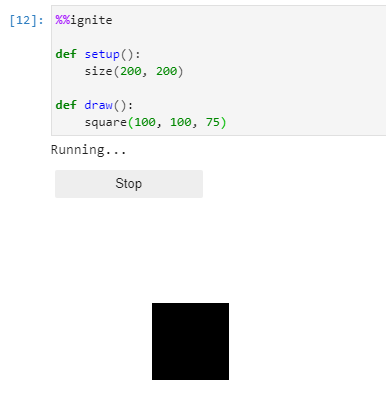#### fill_square()

 `1` ``````fill_square(x, y, s) ``````

Parameters

• x: (int or float) The value of the x position of the square
• y: (int or float) The value of the y position of the square
• s: (int or float) The width and height of the square

Example(s):

Creating a filled square at (100, 100) with a width and height of 75

 ```1 2 3 4 5 6 7``` ``````%%ignite def setup(): size(200, 200) def draw(): fill_square(100, 100, 75) ``````

Results in: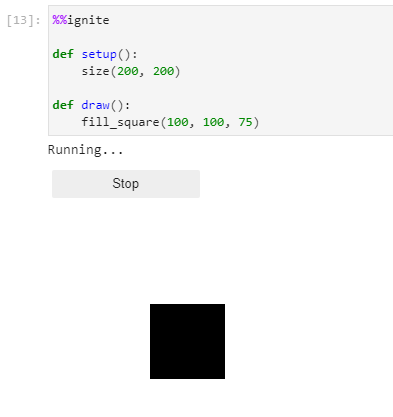#### stroke_square()

 `1` ``````stroke_square(x, y, s) ``````

Parameters

• x: (int or float) The value of the x position of the square
• y: (int or float) The value of the y position of the square
• s: (int or float) The width and height of the square

Example(s):

Creating a stroked square at (100, 100) with a width and height of 75

 ```1 2 3 4 5 6 7``` ``````%%ignite def setup(): size(200, 200) def draw(): stroke_square(100, 100, 75) ``````

Results in: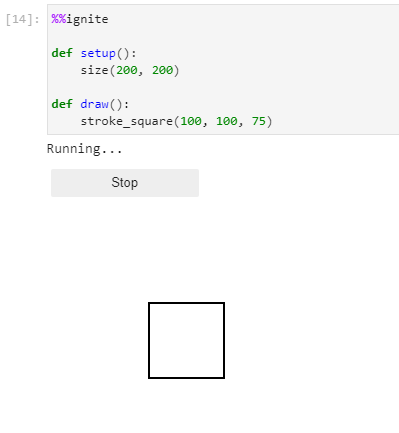### Circles

To create a circle there are 3 options:

Function Description
circle(x, y, d) Draw a circle filled with color, and with a stroke
fill_circle(x, y, d) Draw an circle filled with a color
stroke_circle(x, y, d) Draw the circle of an ellipse (it's stroke)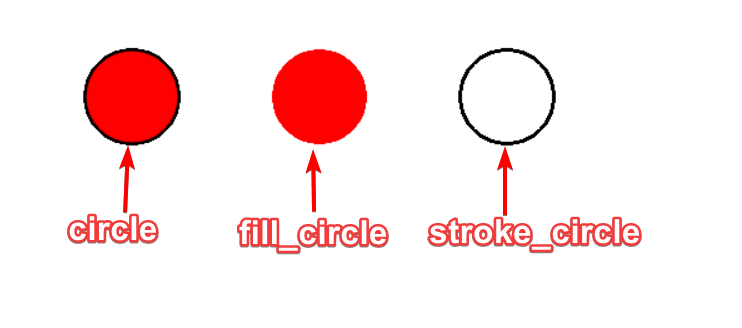Comparison of 3 functions, note the red color was added for visibility, by default fill is black

#### circle()

 `1` ``````circle(x, y, d) ``````

Parameters

• x: (int or float) The value of the x position of the rectangle
• y: (int or float) The value of the y position of the rectangle
• d: (int or float) The diameter of the circle

Example(s):

Creating a filled and stroked circle at (100, 100) with a diameter of 75

 ```1 2 3 4 5 6 7``` ``````%%ignite def setup(): size(200, 200) def draw(): circle(100, 100, 75) ``````

Results in: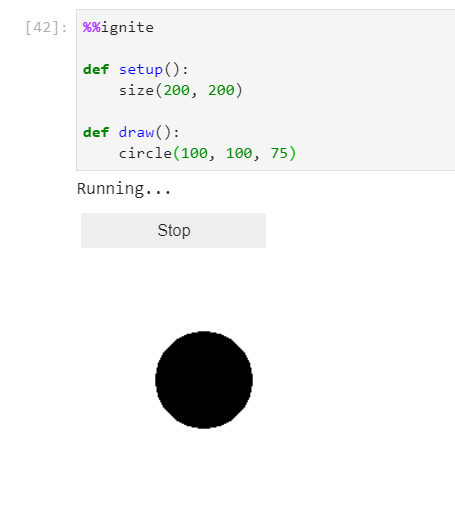#### fill_circle()

 `1` ``````fill_circle(x, y, d) ``````

Parameters

• x: (int or float) The value of the x position of the rectangle
• y: (int or float) The value of the y position of the rectangle
• d: (int or float) The diameter of the circle

Example(s):

Creating a filled circle at (100, 100) with a diameter of 75

 ```1 2 3 4 5 6 7``` ``````%%ignite def setup(): size(200, 200) def draw(): fill_circle(100, 100, 75) ``````

Results in: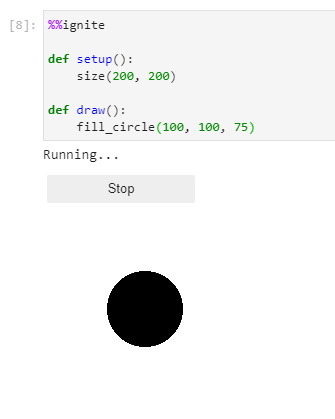#### stroke_circle()

 `1` ``````stroke_circle(x, y, d) ``````

Parameters

• x: (int or float) The value of the x position of the rectangle
• y: (int or float) The value of the y position of the rectangle
• d: (int or float) The diameter of the circle

Example(s):

Creating a sroked circle at (100, 100) with a diameter of 75

 ```1 2 3 4 5 6 7``` ``````%%ignite def setup(): size(200, 200) def draw(): stroke_circle(100, 100, 75) ``````

Results in:### Ellipses

To create an ellipse there are 3 options:

Function Description
ellipse(x, y, l, w) Draw an ellipse filled with color, and with a stroke
fill_ellipse(x, y, l, w) Draw an ellipse filled with a color
stroke_ellipse(x, y, l, w) Draw the outline of an ellipse (it's stroke)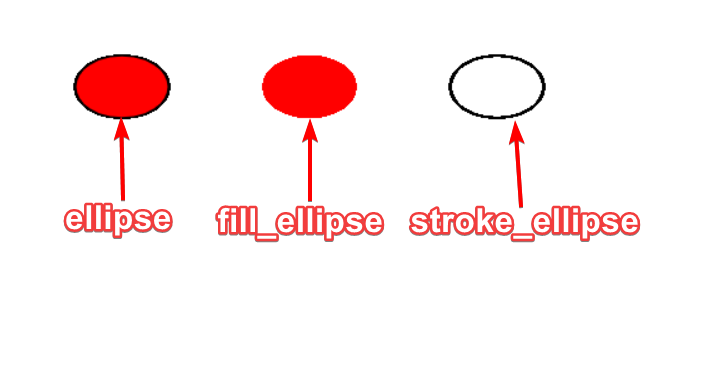Comparison of 3 functions, note the red color was added for visibility, by default fill is black

#### ellipse()

 `1` ``````ellipse(x, y, l, w) ``````

Parameters

• x: (int or float) The value of the x position of the rectangle
• y: (int or float) The value of the y position of the rectangle
• l: (int or float) The length of the ellipse
• w: (int or float) The width of the ellipse

Example(s):

Creating a filled and stroked ellipse at (100, 100) with a length of 100 and height of 75

 ```1 2 3 4 5 6 7``` ``````%%ignite def setup(): size(200, 200) def draw(): ellipse(100, 100, 100, 75) ``````

Results in:#### fill_ellipse()

 `1` ``````fill_ellipse(x, y, l, w) ``````

Parameters

• x: (int or float) The value of the x position of the rectangle
• y: (int or float) The value of the y position of the rectangle
• l: (int or float) The length of the ellipse
• w: (int or float) The width of the ellipse

Example(s):

Creating a filled ellipse at (100, 100) with a length of 100 and height of 75

 ```1 2 3 4 5 6 7``` ``````%%ignite def setup(): size(200, 200) def draw(): fill_ellipse(100, 100, 100, 75) ``````

Results in: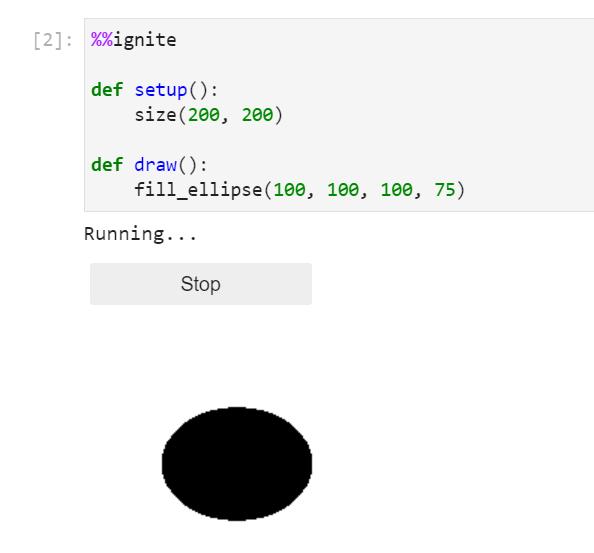#### stroke_ellipse()

 `1` ``````stroke_ellipse(x, y, l, w) ``````

Parameters

• x: (int or float) The value of the x position of the rectangle
• y: (int or float) The value of the y position of the rectangle
• l: (int or float) The length of the ellipse
• w: (int or float) The width of the ellipse

Example(s):

Creating a filled ellipse at (100, 100) with a length of 100 and height of 75

 ```1 2 3 4 5 6 7``` ``````%%ignite def setup(): size(200, 200) def draw(): stroke_ellipse(100, 100, 100, 75) ``````

Results in: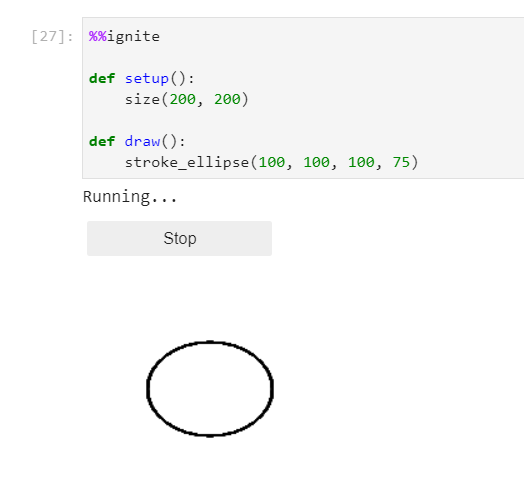### Triangles

To create a triangle there are 3 options:

Function Description
traingle(x1, y1, x2, y2, x3, y3) Draw a triangle filled with color, and with a stroke
fill_triangle(x1, y1, x2, y2, x3, y3) Draw a triangle filled with a color
stroke_triangle(x1, y1, x2, y2, x3, y3) Draw the outline of a trianlge (it's stroke)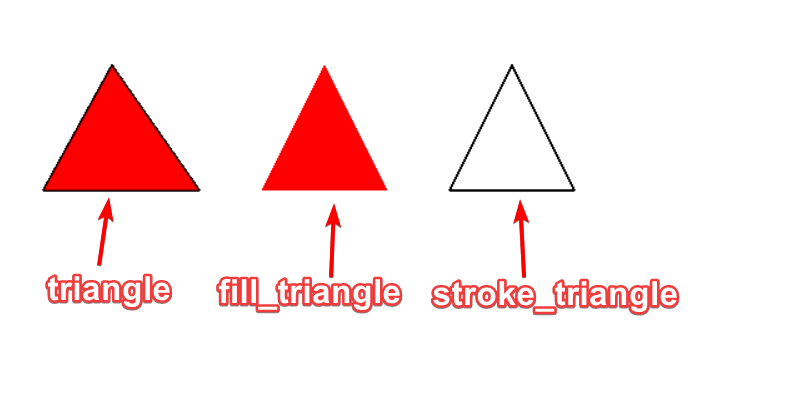Comparison of 3 functions, note the red color was added for visibility, by default fill is black

#### triangle()

 `1` ``````triangle(x1, y1, x2, y2, x3, y3) ``````

Parameters

• x1: (int or float) The value of the x position first of the three points
• y1: (int or float) The value of the y position first of the three points
• x2: (int or float) The value of the x position second of the three points
• y2: (int or float) The value of the y position second of the three points
• x3: (int or float) The value of the x position last of the three points
• y3: (int or float) The value of the y position last of the three points

Example(s):

Creating a triangle with points at (110, 75), (75, 150), (150, 150)

 ```1 2 3 4 5 6 7``` ``````%%ignite def setup(): size(200, 200) def draw(): triangle(110, 75, 75, 150, 150, 150) ``````

Results in: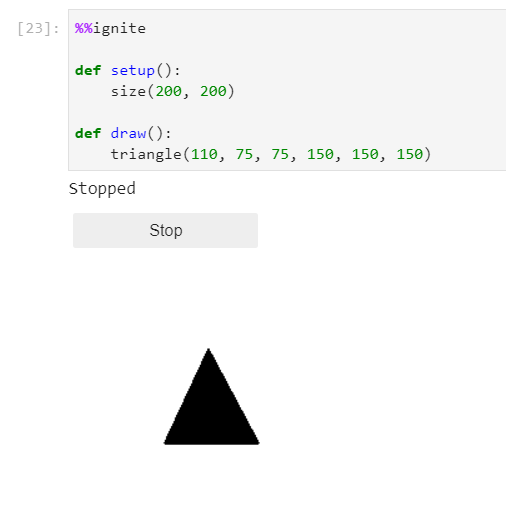#### fill_triangle()

 `1` ``````fill_triangle(x1, y1, x2, y2, x3, y3) ``````

Parameters

• x1: (int or float) The value of the x position first of the three points
• y1: (int or float) The value of the y position first of the three points
• x2: (int or float) The value of the x position second of the three points
• y2: (int or float) The value of the y position second of the three points
• x3: (int or float) The value of the x position last of the three points
• y3: (int or float) The value of the y position last of the three points

Example(s):

Creating a filled triangle with points at (110, 75), (75, 150), (150, 150)

 ```1 2 3 4 5 6 7``` ``````%%ignite def setup(): size(200, 200) def draw(): fill_triangle(110, 75, 75, 150, 150, 150) ``````

Results in: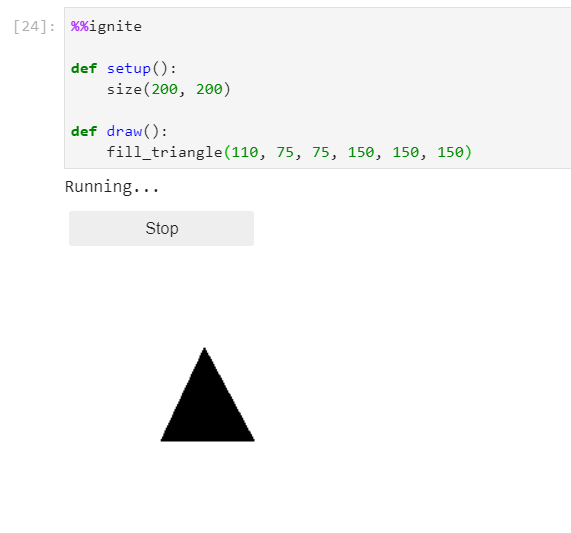#### stroke_triangle()

 `1` ``````stroke_triangle(x1, y1, x2, y2, x3, y3) ``````

Parameters

• x1: (int or float) The value of the x position first of the three points
• y1: (int or float) The value of the y position first of the three points
• x2: (int or float) The value of the x position second of the three points
• y2: (int or float) The value of the y position second of the three points
• x3: (int or float) The value of the x position last of the three points
• y3: (int or float) The value of the y position last of the three points

Example(s):

Creating a stroked triangle with points at (110, 75), (75, 150), (150, 150)

 ```1 2 3 4 5 6 7``` ``````%%ignite def setup(): size(200, 200) def draw(): stroke_triangle(110, 75, 75, 150, 150, 150) ``````

Results in: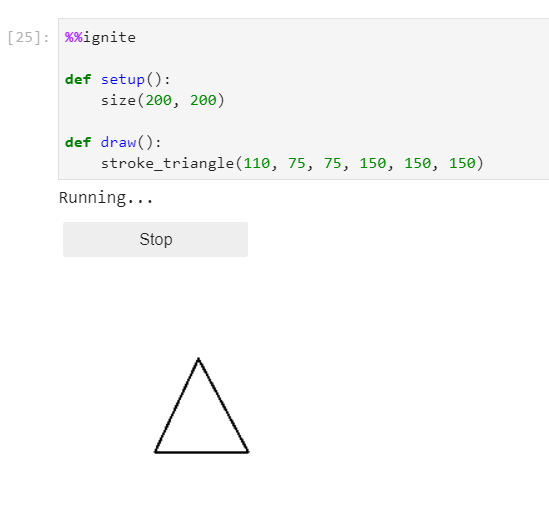### Text

To create text in your drawing use:

 `1` ``````text(message, x, y) ``````

Parameters

• message: (str) The text you want to draw
• x: (int or float) The value of the x position of the text
• y: (int or float) The value of the y position of the text

Example(s):

Creating some text at (100, 100)

 ```1 2 3 4 5 6 7``` ``````%%ignite def setup(): size(200, 200) def draw(): text("Hello World!", 100, 100) ``````

Results in: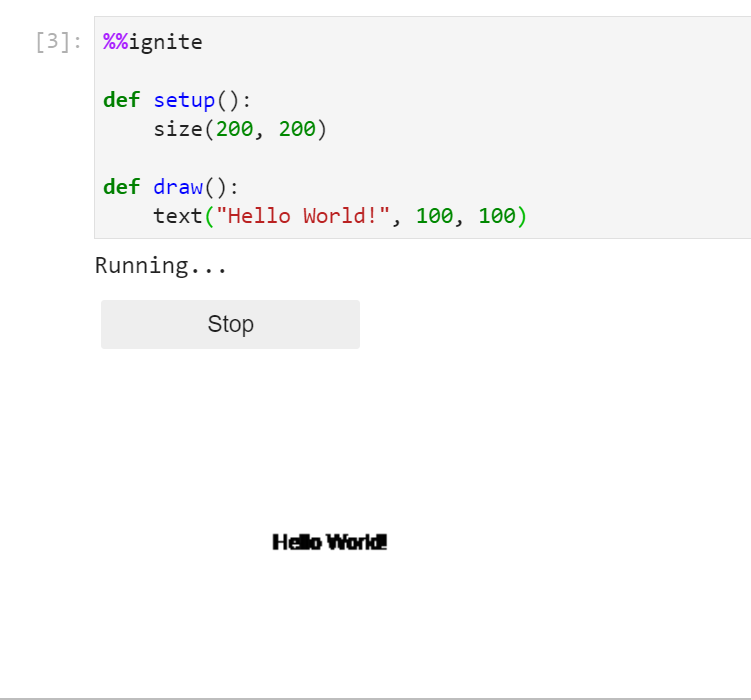#### Change text size

To change the size of your text use:

 `1` ``````text_size(s) ``````

Parameters

• s: (int or float) The size you want to make your text

Example(s):

Creating some text at (100, 100), that is 16pt font

 ```1 2 3 4 5 6 7 8``` ``````%%ignite def setup(): size(200, 200) def draw(): text_size(16) text("Hello World!", 100, 100) ``````

Results in: